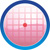2. ALGEBRA
2.1 Introduction(Constants, Variables, Degree, Monomial, Binomial, Trinomial, Polynomial) 8 8 8,9 9 9 9
2.2 Exponents:(Base, Power, Law of exponents) 8 8 8,9 8   8 9
2.3 Logarithm( Laws of Logarithm, Antilogarithm)     8,9     9 9
2.4 Multiplication of algebraic expressions(Monomial,Binomial,Identities (algebraic formulae) 8 8 8,9 8,9 9 8 9
2.5 HCF(GCD) and LCM of algebraic terms 8   8,9
2.6 Factorisation of trinomials 8 8 8,9 8   8 9
2.7 Linear equations(Axioms, Solving Linear equations) 8 8 8,9 8,9     8
2.8 Factorisation of algebraic expressions (using identities, Product of three binomials) 9 9 8,9 8,9   8 10
2.9 HCF and LCM of Polynomials 9 10 8,9       10
2.10 Division of polynomial by monomial, binomial(Synthetic method,roots) 9 8 8,9 8,9 8,9,10 9,10
2.11 Remainder, Factor theorem 10 9 10 9 10 10 10
2.12 Rational expressions of polynomials 10 10
2.13 Variation 9
2.14 Solving of Simultaneous linear equations( in two,three variables)( in two,three variables) 9 10 8,9 8,10 9 8 8,10
2.15 Algebraic structures (Binary operation, Closure property)(Binary operation, Closure property) 9 8,9
2.16 Cyclic symmetry     10   9
2.17 Conditional identities     9
2.18 Algebraic Surds(Operation on surds, Rationalization) 10
2.19 Quadratic equations(Solving by factorisation, formula methods, roots, co-efficients, formation of equations,Binomial theorem, Pascal triangle) 10 10 10 8,9,10 10 10 10